# Dilated ResNet-22 Trained onCityscapes Data

Segment an image of a driving scenario into semantic component classes

Released in 2017, this architecure combines the technique of dilated convolutions with the paradigm of residual networks, outperforming their nonrelated counterparts in image classification and semantic segmentation.

Number of layers: 86 | Parameter count: 15,994,691 | Trained size: 64 MB |

## Training Set Information

• Cityscapes, a collection of 25,000 annotated images for semantic understanding of urban street scenes.

## Performance

• This model achieves 68% mean IoU accuracy on the Cityscapes dataset.

## Examples

### Resource retrieval

Get the pre-trained net:

 In:=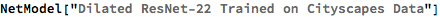Out=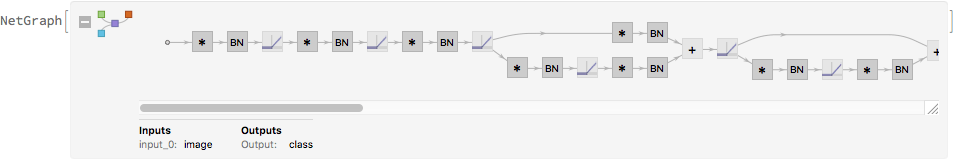### Evaluation function

Write an evaluation function to handle net reshaping and resampling of input and output:

 In:=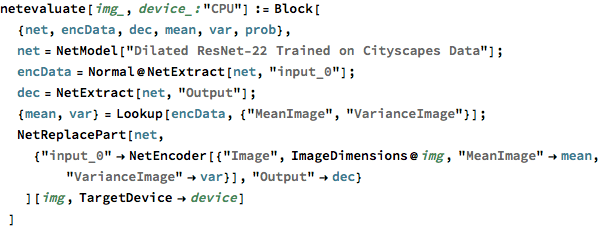### Label list

Define the label list for this model. Integers in the model’s output correspond to elements in the label list:

 In:=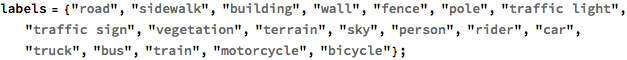### Basic usage

Obtain a segmentation mask for a given image:

 In:=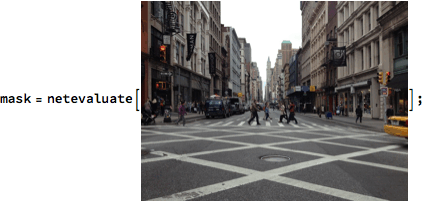Inspect which classes are detected:

 In:=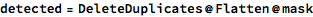Out=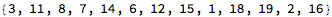In:=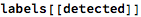Out=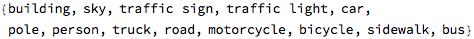In:=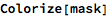Out=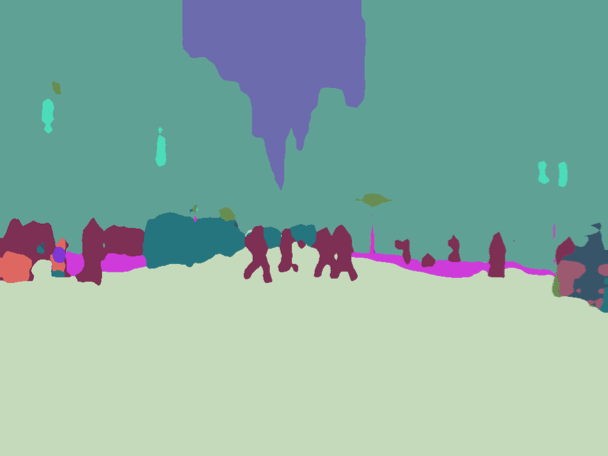Associate classes to colors using the standard Cityscapes palette:

 In:=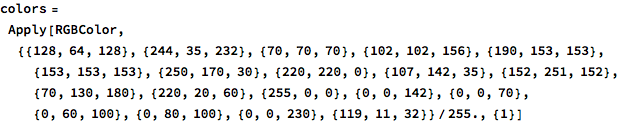Out=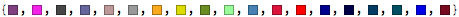In:=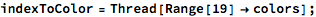Write a function to overlap the image and the mask with a legend:

 In:=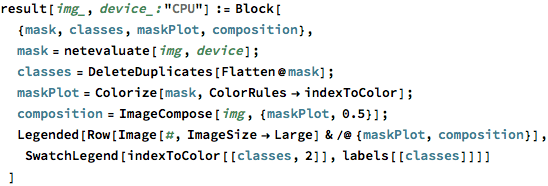Inspect the results:

 In:=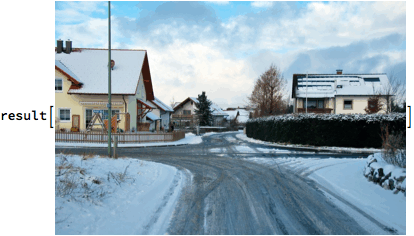Out=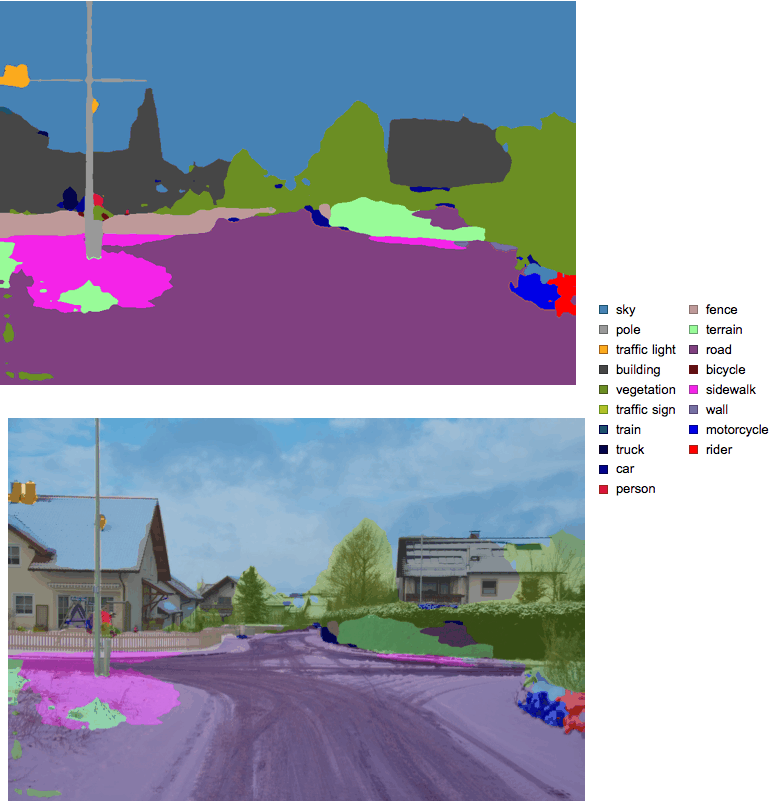In:=Out=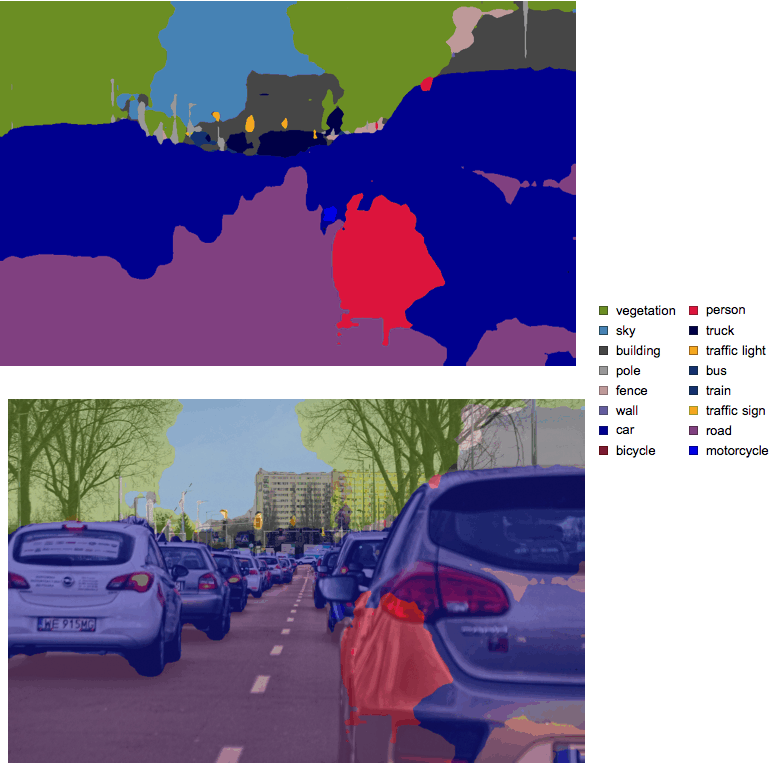In:=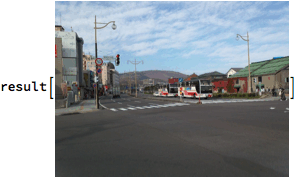Out=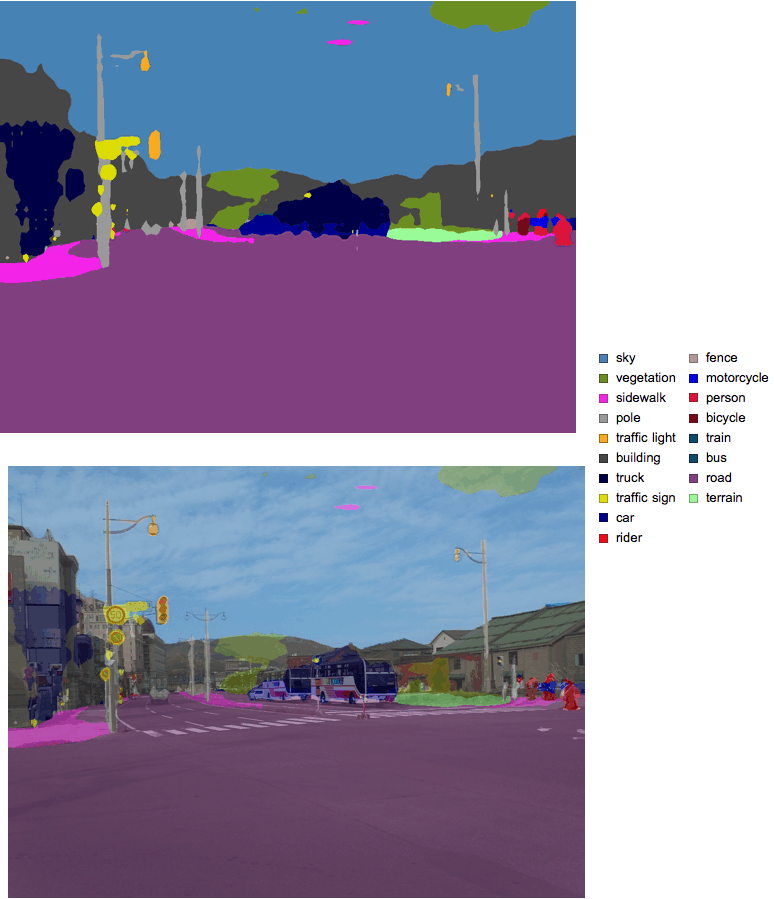### Net information

Inspect the number of parameters of all arrays in the net:

 In:=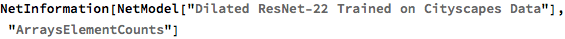Out=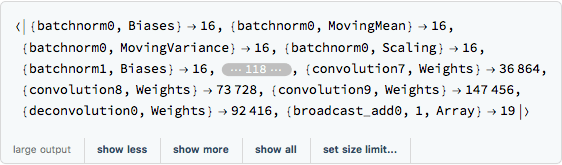Obtain the total number of parameters:

 In:=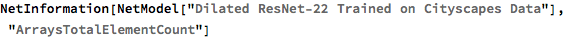Out=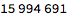Obtain the layer type counts:

 In:=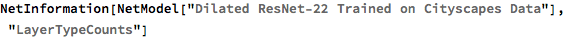Out=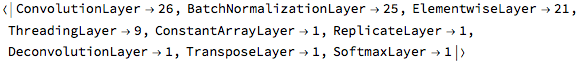Display the summary graphic:

 In:=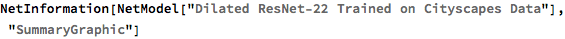Out=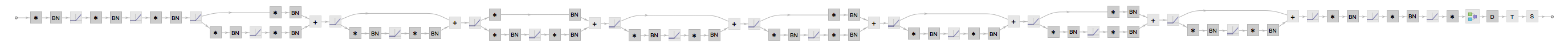### Export to MXNet

Export the net into a format that can be opened in MXNet:

 In:=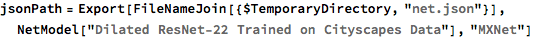Out=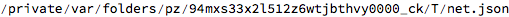Export also creates a net.params file containing parameters:

 In:=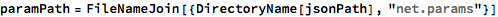Out=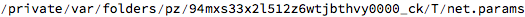Get the size of the parameter file:

 In:=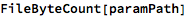Out=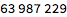The size is similar to the byte count of the resource object:

 In:=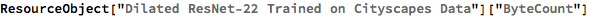Out=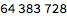Represent the MXNet net as a graph:

 In:=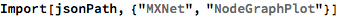Out=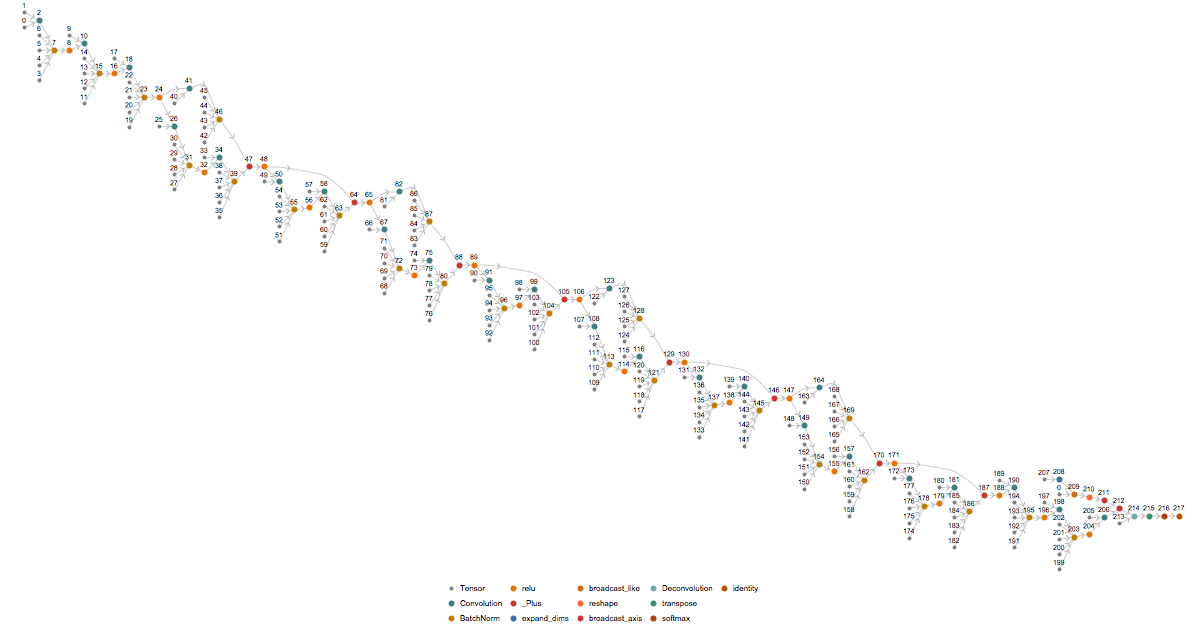## Requirements

Wolfram Language 11.3 (March 2018) or above